# How to Use sum() Function in R

The sum() function in R is used to calculate the sum of all the values present in its arguments.

## Syntax

``sum(x, na.rm = FALSE)``

## Parameters

1. x: It is a numeric vector or data frame.
2. na.rm: whether NA should be removed; if not, NA will be returned.

## Example 1: Using sum() with vector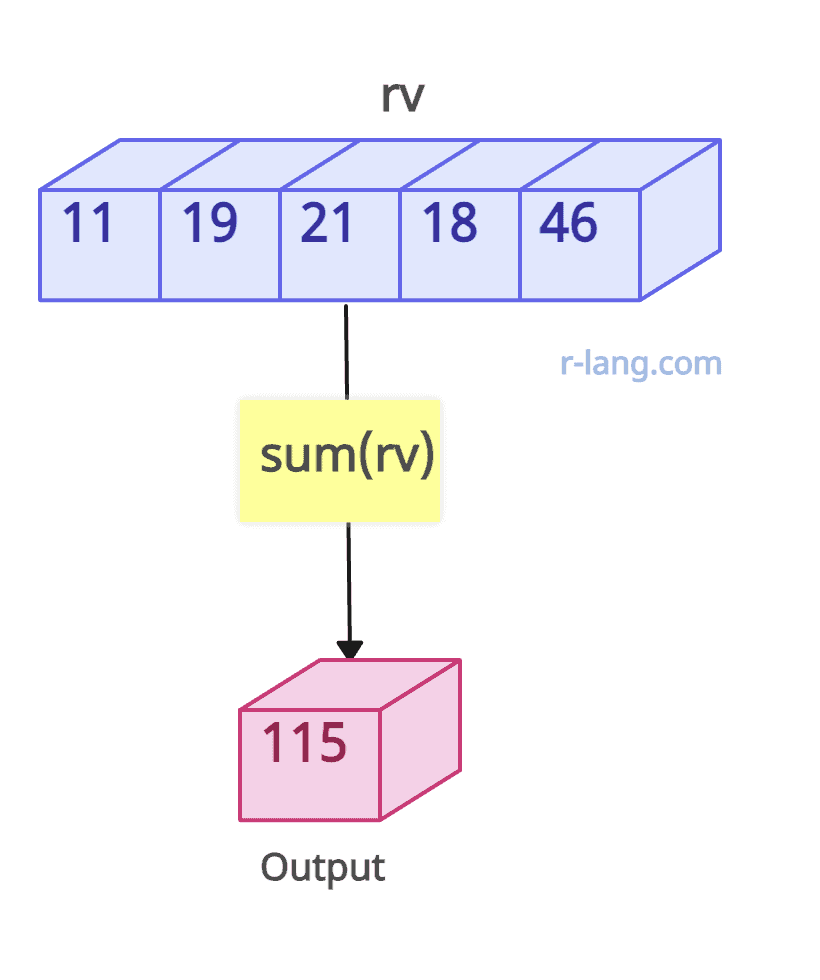``````rv <- c(11, 19, 21, 18, 46)

# Calculates the sum of the values
sum(rv)``````

Output

`` 115``

You can see that we calculated the sum of all vector elements using the sum() function.

## Example 2: Passing the NA value

You can’t get the desired output if NA is present in the vector elements.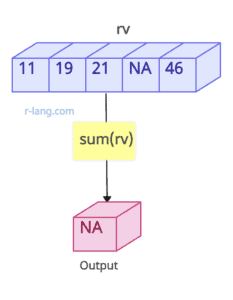``````vec <- c(11, 19, 21, NA, 46)

# Calculates the sum of rv vector
sum(vec)
``````

Output

`````` NA
``````

Well, we did not expect NA output. However, sometimes your dataset may contain ‘NA” values, i.e., ‘Not Available’. But we can handle this issue by bypassing na.rm = TRUE.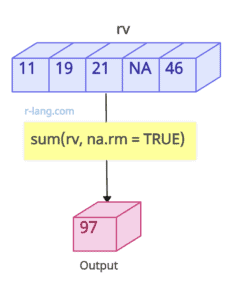``````vec <- c(11, 19, 21, NA, 46)

# Calculates the sum of the values
sum(vec, na.rm = TRUE)``````

Output

`` 97``

## Example 3: Using sum() function with complete data frame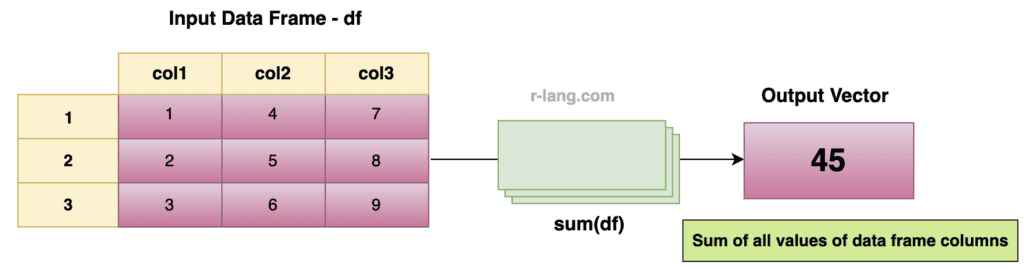``````df <- data.frame(
col1 = c(1, 2, 3),
col2 = c(4, 5, 6),
col3 = c(7, 8, 9)
)

sum(df)
``````

Output

`````` 45
``````

We calculated the sum of all the elements in the data frame using the sum(df) function, adding the values in all three columns.

## Example 4: Adding values of a specific column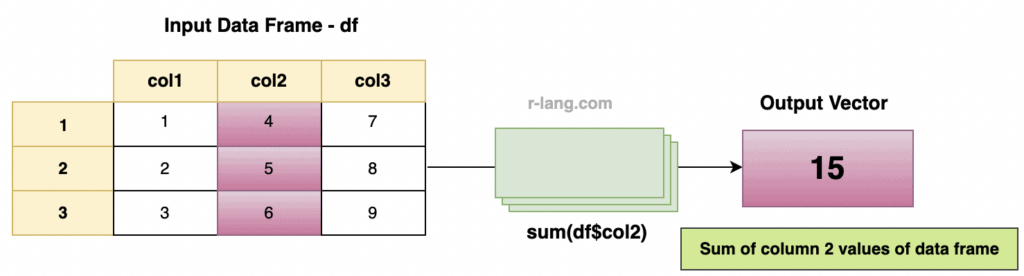``````df <- data.frame(
col1 = c(1, 2, 3),
col2 = c(4, 5, 6),
col3 = c(7, 8, 9)
)

sum(df\$col2)
``````

To access the column, use \$ and then the column name. Here we are finding the sum of enrollno column values. See the below output.

`````` 15
``````

## Example 5: Adding values of multiple columns

To get the sum of multiple columns, use the “mapply()” function in combination with the sum() function.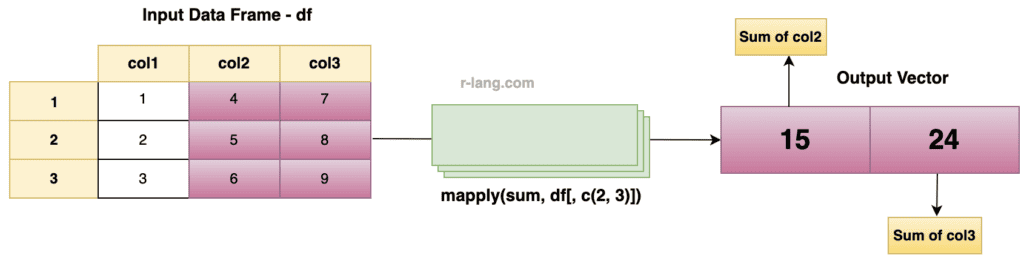``````df <- data.frame(
col1 = c(1, 2, 3),
col2 = c(4, 5, 6),
col3 = c(7, 8, 9)
)

mapply(sum, df[, c(2, 3)]) ``````

Output

``````col2   col3
15     24
``````

That’s it.